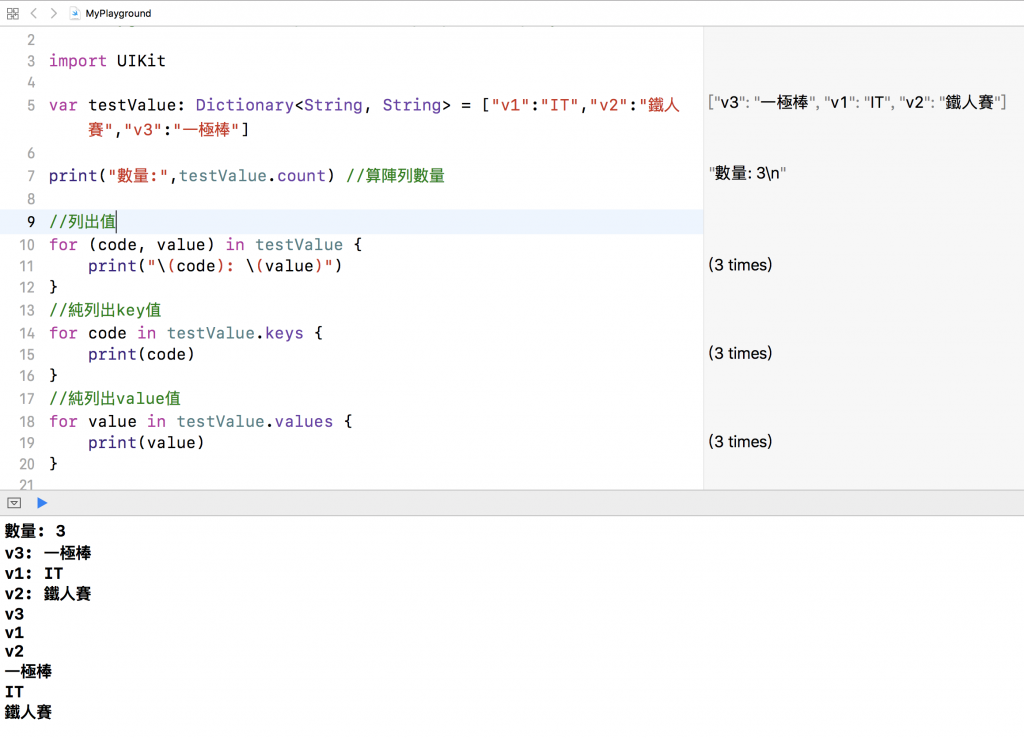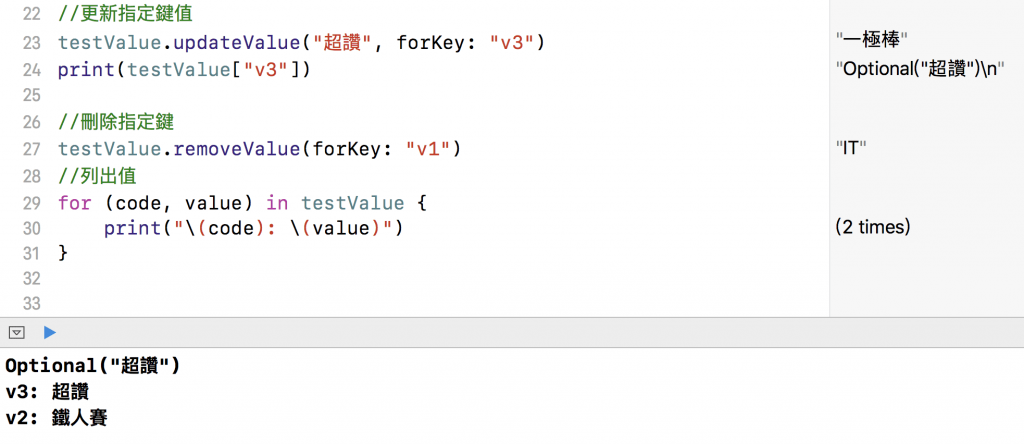#2019 iT 邦幫忙鐵人賽DAY 5
1

## [2019鐵人賽Day5]老蕭咖啡館-平淡無奇的基礎觀念說明(下)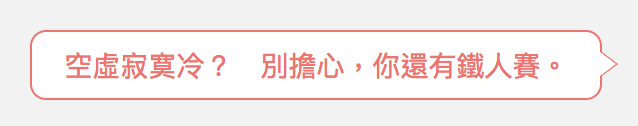==========

Swift迴圈有：while、repeat-while、for-in

「while迴圈」

``````while 條件運算式 {
迴圈執行的程式敘述
}
``````

while迴圈敘述要先判斷條件運算式是否為真

Example:

``````var total = 0, index = 1
while index <= 32{
total += index
index += 1
}
print("1加到32的值為：\(total)")
``````

print("1加到32再減8的值為我想對妳說的話：(total-8)")

[過段片刻]

「repeat-while迴圈」
repeat-while迴圈會先執行一次程式，再檢查條件運算式，接著循迴圈執行程式直到條件運算式返回false

``````repeat {
迴圈執行的程式
} while 條件運算式
``````

Example:

``````var total = 0,index = 1
repeat {
total += index
index += 1
}while index <= 32
print("1加到32的值為：\(total)")
print("1加到32再減8的值為我想對妳說的話：\(total-8)")
``````

「for-in迴圈」

``````for item in items {
迴圈執行的程式敘述
}
``````

for-in迴圈，會遍歷一個集合內的所有項目，像是一個數字區間、陣列、字典中的值之類

Example:

``````var total = 0
for n in 1...32 {
total += n
}
print("1加到32的值為：\(total)")
print("1加到32再減8的值為我想對妳說的話：\(total-8)")
``````

「巢狀迴圈(nested loop)」

Example:

``````// 九九乘法表
for i in 1...9 {
for j in 1...9 {
print("\(i) * \(j) = \(i*j)")
}
print("\r")
}
``````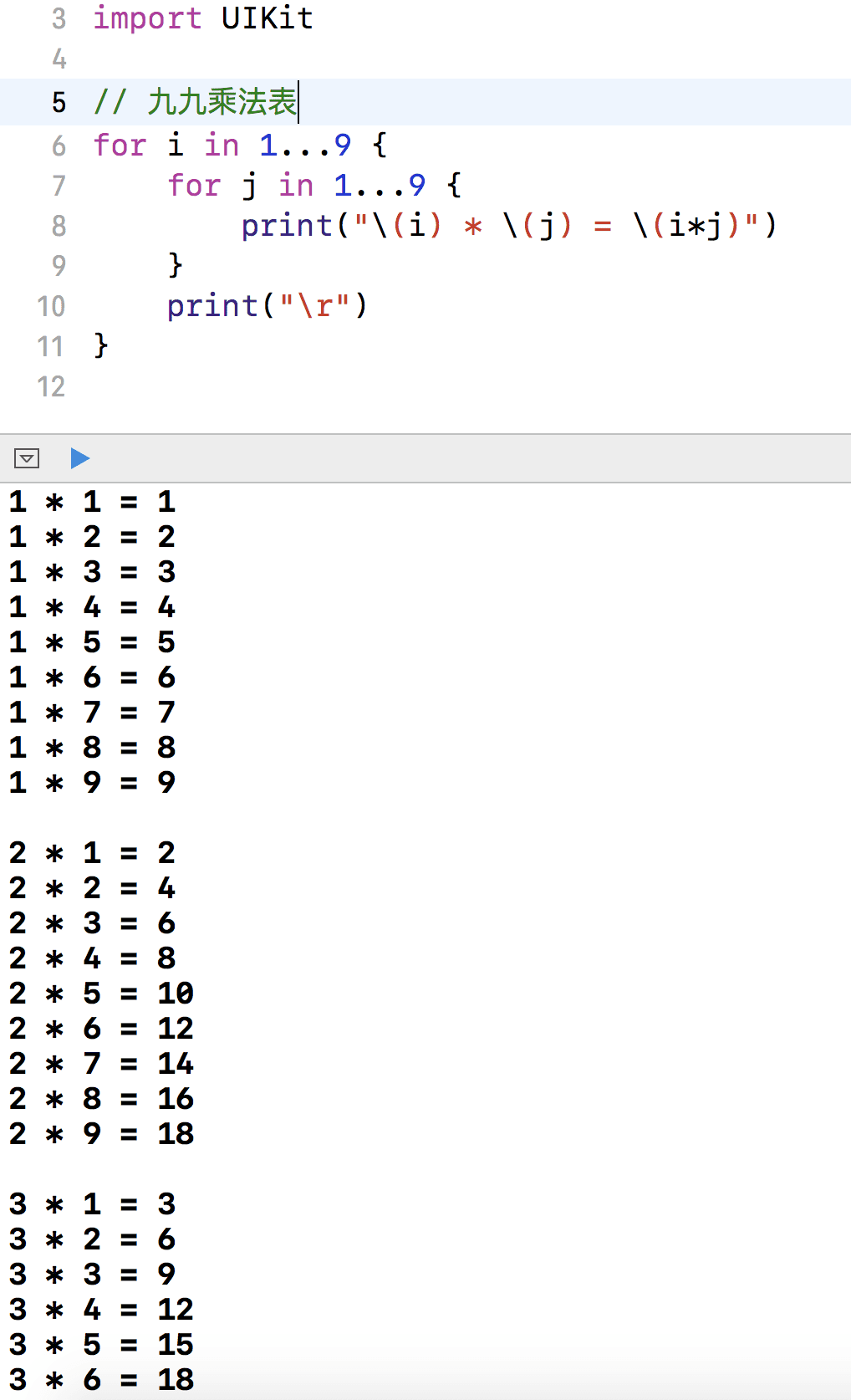==========

「集合型別」
Swift提供三種基本的集合型別：Array、Set、Dictionary 用來儲存集合資料

Array陣列：按順序儲存資料
Set集合：沒有順序，不能重複儲存資料
Dictionary字典：沒有順序，key : value（識別鍵 : 值），經由唯一的識別鍵找到需要的值

「陣列」

Example:

var v1: [String] = []

var v1 = ["Go","Good"]
or
var v1: [String] = ["Go","Good"]

Example:

``````var testValue = ["IT","鐵人賽","一極棒"]
for valueOutput in testValue {
print(valueOutput)
}
``````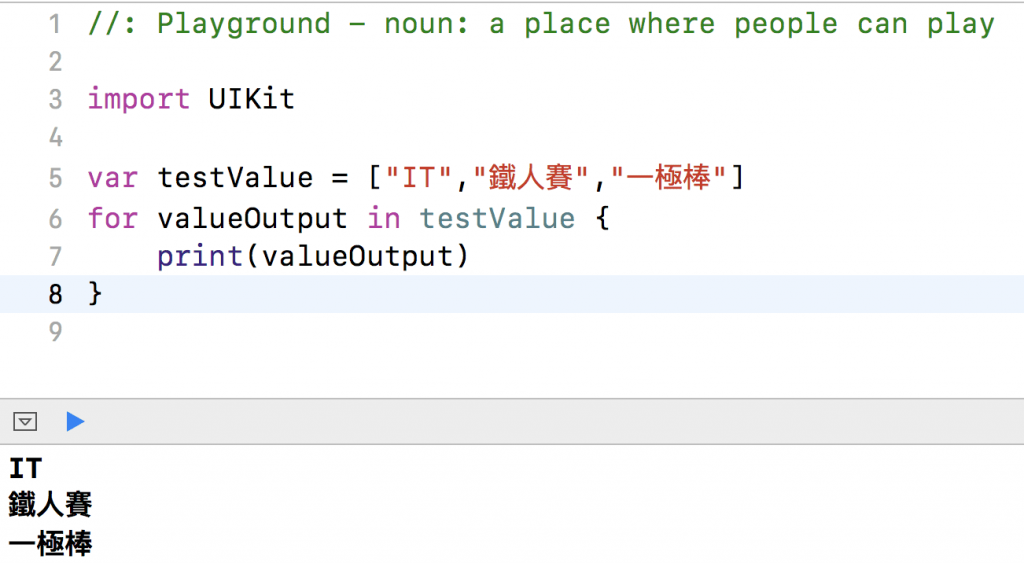「集合型別」
Swift提供三種基本的集合型別：Array、Set、Dictionary 用來儲存集合資料

Array陣列：按順序儲存資料
Set集合：沒有順序，不能重複儲存資料
Dictionary字典：沒有順序，key : value（識別鍵 : 值），經由唯一的識別鍵找到需要的值

「陣列」

Example:

``````var v1: [String] = []
``````

var v1 = ["Go","Good"]
or
var v1: [String] = ["Go","Good"]

Example:

``````var testValue = ["IT","鐵人賽","一極棒"]
for valueOutput in testValue {
print(valueOutput)
}
``````

count是用來表示陣列內值的數量
isEmpty是用來檢查陣列內是否有值，並會返回一個布林值
insert是用來把指定值加入指定陣列位置
removeAtIndex(x)是用來指定位置資料
removeLast是用來刪除最後一筆資料

Example:

``````var testValue = ["IT","鐵人賽","一極棒"]
testValue.count //算陣列數量
testValue.insert("超讚", at: 3) //加入指定位置
testValue.remove(at: 0) //刪除指定位置
testValue.removeLast() //刪除最後一筆

for valueOutput in testValue {
print(valueOutput)
}
``````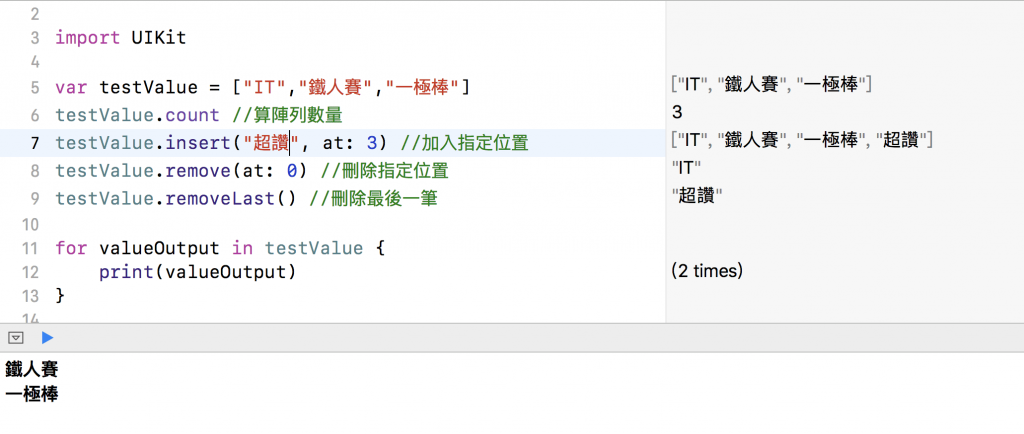「字典」

var dict: Dictionary<鍵的型別, 值得型別>
or
var dict: [鍵的型別: 值的型別]

updateValue(value, forKey: key)

removeValue(forKey: key)

Example:

``````var testValue: Dictionary<String, String> = ["v1":"IT","v2":"鐵人賽","v3":"一極棒"]

print("數量:",testValue.count) //算陣列數量

//列出值
for (code, value) in testValue {
print("\(code): \(value)")
}
//純列出key值
for code in testValue.keys {
print(code)
}
//純列出value值
for value in testValue.values {
print(value)
}

//更新指定鍵值
testValue.updateValue("超讚", forKey: "v3")
print(testValue["v3"])

//刪除指定鍵
testValue.removeValue(forKey: "v1")
//列出值
for (code, value) in testValue {
print("\(code): \(value)")
}
``````